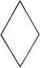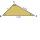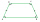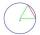# Rhombus 29

One of the diagonals of a rhombus is equal to a side of the rhombus. Find the angles of the rhombus.

Result

A =  120 °
B =  60 °
C =  120 °
D =  60 °

#### Solution:Leave us a comment of example and its solution (i.e. if it is still somewhat unclear...):Be the first to comment!## Next similar examples:

1. DiagonalCan a rhombus have the same length diagonal and side?
2. DiagonalCan be a diagonal of diamond twice longer than it side?
3. Rhombus anglesIf one angle in the rhombus is 159°, what is it neighboring angle in rhombus?
4. Type of triangleHow do I find the triangle type if the angle ratio is 2:3:7 ?
5. Plane IIA plane flew 50 km on a bearing 63degrees20 and the flew on a bearing 153degrees20 for 140km. Find the distance between the starting point and the ending point
6. MO 2016 Numerical axisCat's school use a special numerical axis. The distance between the numbers 1 and 2 is 1 cm, the distance between the numbers 2 and 3 is 3 cm, between the numbers 3 and 4 is 5 cm and so on, the distance between the next pair of natural numbers is always in
7. Isosceles trapezoid v3In an isosceles trapezoid ABCD is the size of the angle β = 81° Determine size of angles α, γ and δ.
8. Angles of a triangleIn the triangle ABC, the angle beta is 15° greater than the angle alpha. The remaining angle is 30° greater than the sum of the angles alpha and beta. Calculate the angles of a triangle.
9. Outer anglesThe outer angle of the triangle ABC at the A vertex is 71°40 ' outer angle at the vertx B is 136°50'. What size has the inner triangle angle at the vertex C?
10. ThomasThomas lives 400 meters away from Samko, Robo from Thomas also 400 m and Samko from Robo 500. Anton lives 300 meters away from Robo further as Samko. How far away lives Anton from Rob?
11. FactoryIn the factory workers work in three shifts. In the first inning operates half of all employees in the second inning and a third in the third inning 200 employees. How many employees work at the factory?
12. NumberWhat number is 20 % smaller than the number 198?
13. Obtuse angleWhich obtuse angle is creating clocks at 17:00?
14. ChordPoint on the circle is the end point of diameter and end point of chord length of radius. What angle between chord and diameter?Convert magnitude of the angle α = 9°39'15" to radians:It is true that the middle traverse bisects the triangle?Construct a triangle ABC is is given c = 60mm hc = 40 mm and b = 48 mm analysis procedure steps construction